Diamond Matrix Beading Pattern Introduction Setting up a 3 Bead Diamond Matrix Rules for Setting Up a Beaded Diamond Matrix Table of Values Setting Up a Beaded Diamond Matrix Planning a Matrix Based Beading Pattern "Download Openface Master Grid"

 ``` Diamond Matrix Beading Pattern A diamond matrix can be used to generate geometric beading patterns for openface fingerweaving. A diamond matrix is created by stringing beads on a selected number of strands. In the example, there is a total of 18 blue strands 6 of these strand are carrier strands for the beads. The total number of strands must be a even number and a multiple of the number of beads on each side of the beaded diamonds in the matrix. The example is a 3 bead matrix. To determine which strands are the carrier strands, start at the middle. The 2 middle strands are used as carrier strands. When the shed is picked up, the shed divides the stands into two layers. One layer is worked on a diagonal from the left to the right, the other is worked on a diagonal from the right to the left. In each layer, the third strand from each of the middle carrier strands becomes a carrier strand. ``` 3 bead diamond matrix carrier strands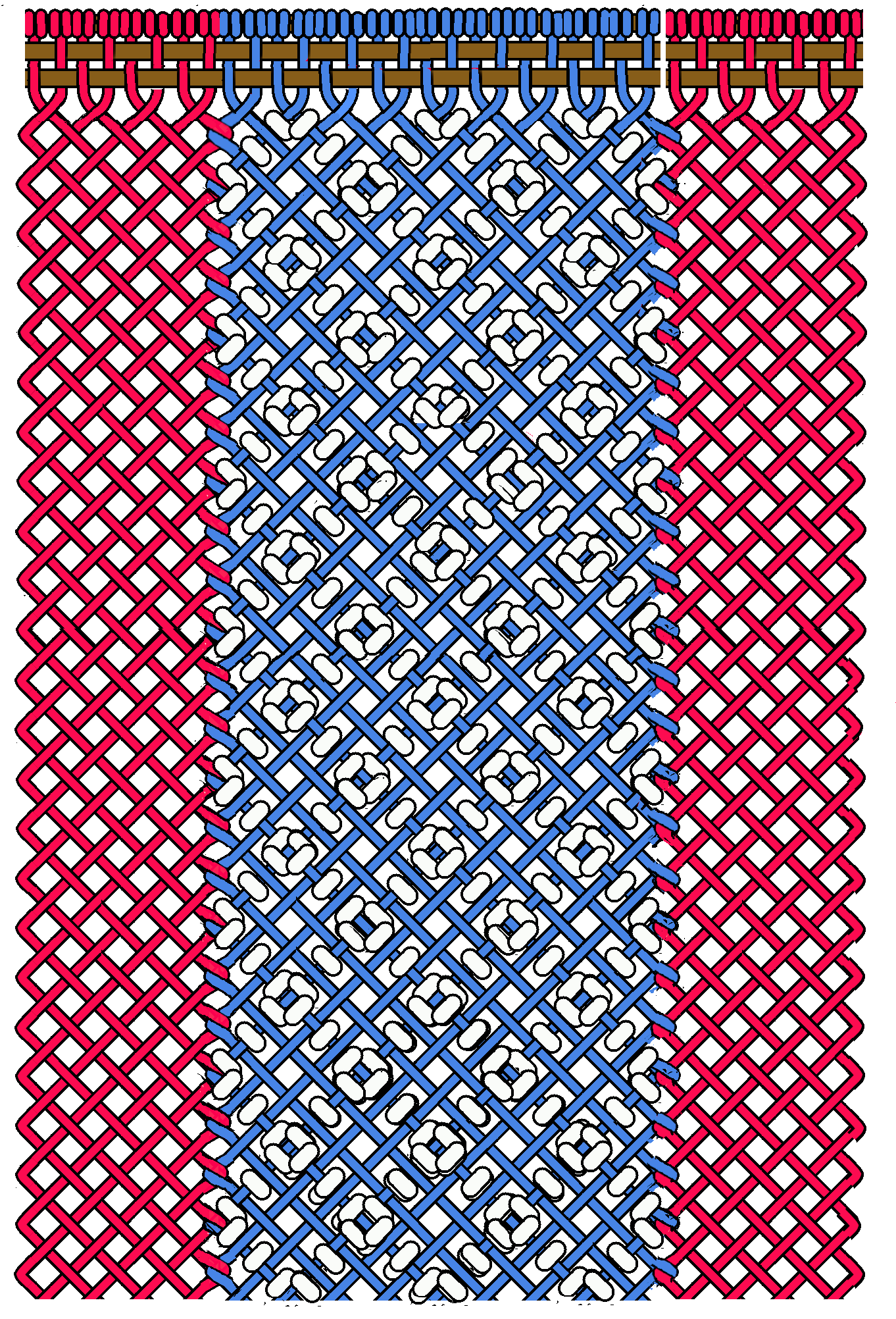<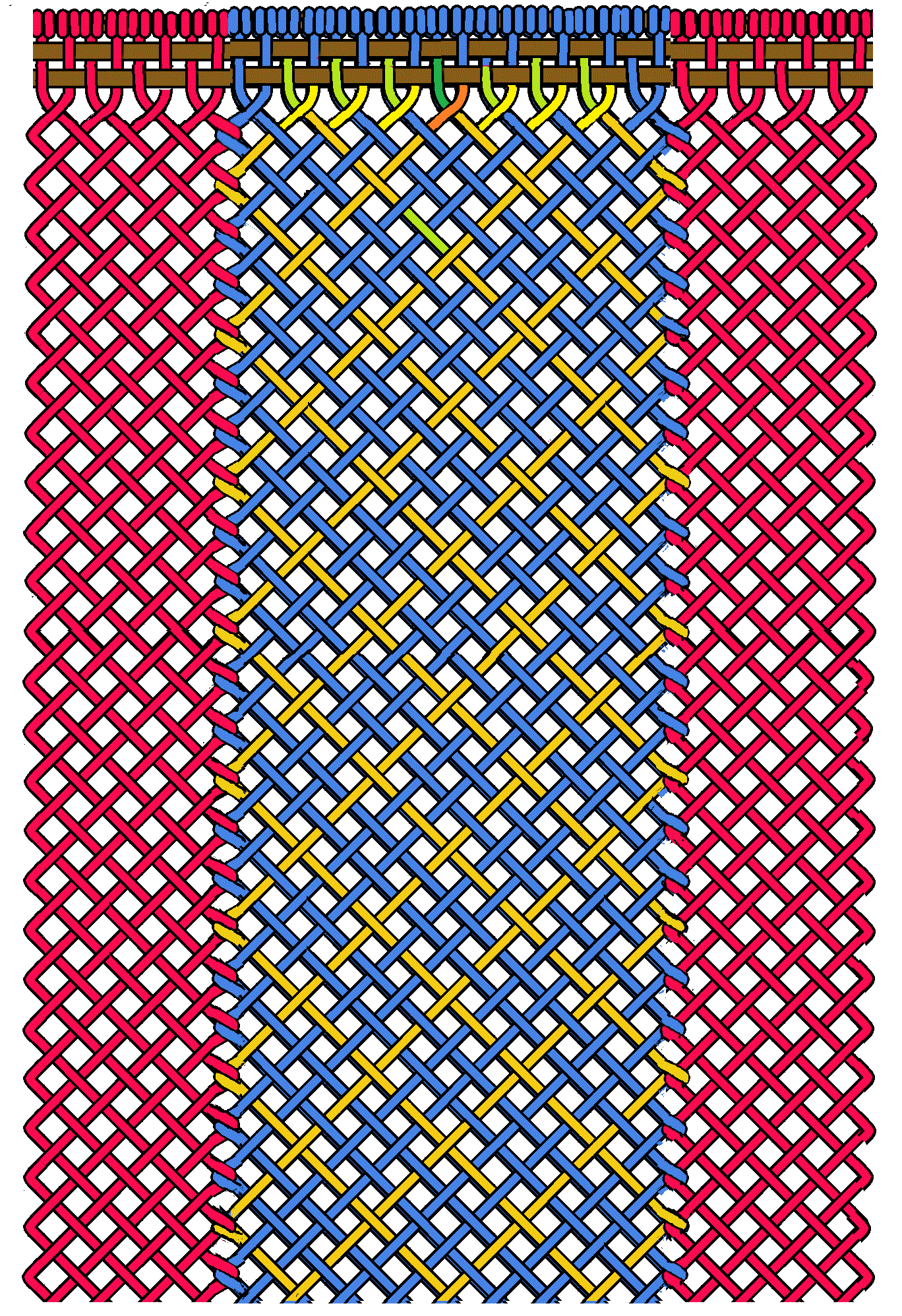Setting up a 3 bead diamond matrix ``` Setting up a 3 bead diamond matrix follows a set of rules. The following graphics will be used to develope and illustrate how to apply these rules. ``` 6 strand 3 bead matrix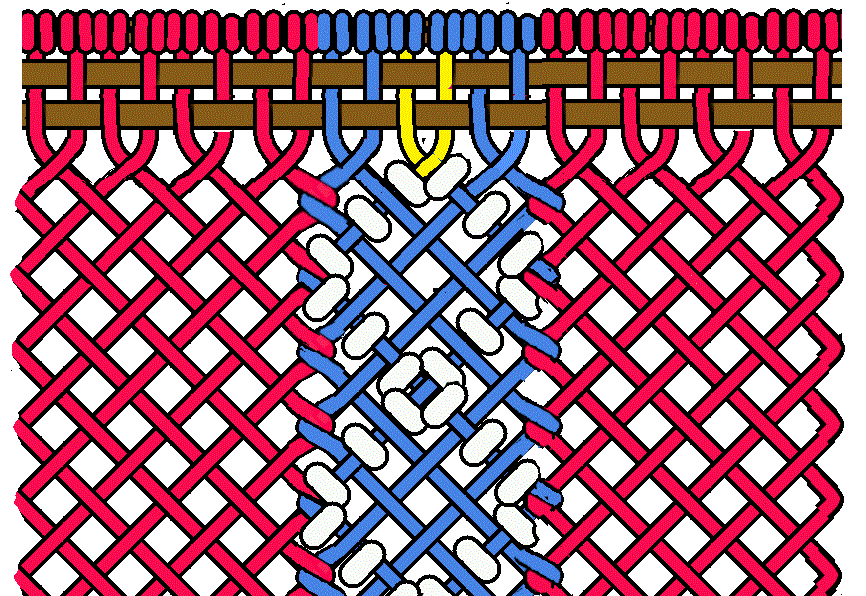``` The 6 strand 3 bead matrix has only 2 carrier strands. This is because there is only 1 strand on either side of the middle carrier strand in each layer. [note] The middle carrier strands cross each other before the beaded diamond is made. This is because there is an odd number of strands on either side of the middle. ```
 12 strand 3 bead matrix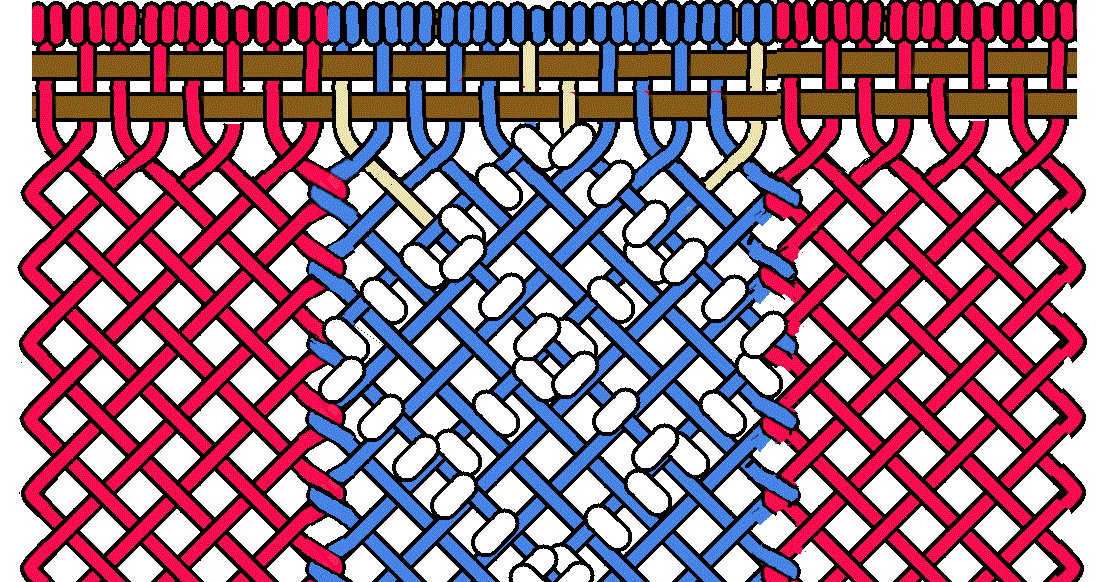``` The next set of strands that is an even number and dividable by 3 is a set of 12 strands. The 12 strand 3 bead matrix has 4 carrier strands. There is only 1 additional carrier strand on either side of the middle carrier strands This is because in each layer there are 3 additional strands on one side and 2 additional strands on the other side of the middle carrier strand in each layer. ```
 ``` [note] The middle carrier strands do not cross each other before the first beaded diamond is made. This is because there is an even number of strands on either side of the middle. ```
 18 strand 3 bead matrix``` The next set of strands that is an even number and a multiple of 3 is a set of 18 strands. The 18 strand 3 bead matrix has 6 carrier strands. There are 2 additional carrier strand on either side of the middle carrier strands This is because in each layer there are more then 3 additional strands on each side of the middle carrier strand in each layer. ```
 ``` [note] The middle carrier strands cross each other before the first beaded diamond is made. This is because there is an odd number of strands on either side of the middle. ```
 24 strand 3 bead matrix``` The next set of strands that is an even number and a multiple of 3 is a set of 24 strands. The 24 strand 3 bead matrix has 8 carrier strands. There are 3 additional carrier strand on either side of the middle carrier strands. This is because on one side of each layer there are 6 strands. 6 is a multiple of 3 three, that allows 2 additional carrier strands on that side. On the other side of the layer there are 5 additional strands, therefore only one additional carrier strand can be added to that the layer. ```
 ``` [note] The middle carrier strands do not cross each other before the first beaded diamond is made. This is because there is an even number of strands on either side of the middle. ```
 Rules for Setting Up a Beaded Diamond Matrix ``` Rule 1; The number of strands in the matrix must be divisible by the number of beads on each edge of the beaded diamond. Rule 2; The number of strands in the matrix must also be an even number. Rule 3; One half the number of strands in the matrix must also be multiple of the number of beads on each edge of the beaded diamond. Rule 4; When identifying the carrier strands, the middle two strand are the first two carrier strands identified. Rule 5; Identify additional carrier strands on either side of the middle carrier strands in each layer of the shed by counting the number of strands equal to the number of beads on each edge of the beaded diamond. One additional carrier strand is added for each set of strands equal to the number of beads on each side of the beaded diamond. [note] If one half the number of strands in the matrix is an odd number, the carrier strand will cross before the first beaded diamond starts. If the number is an even number the the carrier strand will not cross before the first beaded diamond is made. ```
 Table of Values ``` 3 Bead Matrix #strands #carrier 6 2 12 4 18 6 24 8 30 10 36 12 42 14 48 16 54 18 ``` ``` 4 Bead Matrix #strands #carrier 8 2 16 4 24 6 32 8 40 10 48 12 56 14 64 16 72 18 ``` ``` 5 Bead Matrix #strands #carrier 10 2 20 4 30 6 40 8 50 10 60 12 70 14 80 16 90 18 ``` ``` 6 Bead Matrix #strands #carrier 12 2 24 4 36 6 48 8 60 10 72 12 84 14 96 16 108 18 ``` ``` 7 Bead Matrix #strands #carrier 14 2 28 4 42 6 56 8 70 10 84 12 98 14 112 16 126 18 ```
 Setting Up a Beaded Diamond Matrix ``` Setting up several 4 bead diamond matrixs will be used to illustrate how to apply the "rules" and the "table of values". ``` 8 strand 4 bead matrix``` The table "4 Bead Matrix" shows that the smallest number of strands that can be used to set up a 4 bead matrix is set of 8 strand. 8 is a multiple of 4, the number of beads on each side of the beaded diamond. 8 is also an even number. One half of 8 is 4 which is a multiple of 4 the number of beads on each edge of the beaded diamond. The Middle two strands are identified as carrier strands. The table of values indicates only 2 carriervstrands, therefore there are no additional carrier strands. Also, if you count the number of strands in each layer of the shed on either side of the middle carrier strand, you will find the count is less than 4, therefore there are no additional carrier strands. ```
 ``` A set of 12 strands is the next multiple of 4, the number of beads on each edge of the beaded diamond and it is an even number but one half 12, which is equal to 6, is not a multiple of 4. Therefore, the set of 12 strands can not be used as a set of strands for a 4 bead matrix. ```
 16 strand 4 bead matrix``` The table "4 Bead Matrix" shows that the next set of strands that can be used as a 4 bead matrix is a set of 16 strands. 16 is multiple of 4, the number of beads on each side of the beaded diamond. 16 is also an even number. One half of 16 is 8 which is a multiple of 4, the number of beads on each edge of the beaded diamond. The Middle two strands are identified as carrier strands. The table of values indicates only 4 carrier strands, therefore there is one additional carrier strand on each side of the middle carrier strands. If you count the number of strands in each layer of the shed on either side of the middle carrier strand, you will find that on one side there are 4 strands. 4 is a multiple of 4. Therefore one additional carrier strand can be added to that side of the shed. The other side of each layer of the shed has 3 strands. 3 is less than 4 therefore, no additional carrier strands can be added to that side of each layer of the shed. ```
 20 strand 4 bead matrix ``` A set of 20 strands is the next multiple of 4, the number of beads on each edge of the beaded diamond and it is an even number but one half 12, which is equal to 6, 6 is not a multiple of 4. Therefore, the set of 20 strands can not be used as a set of strands for a 4 bead diamond matrix. ```
 24 strand 4 bead matrix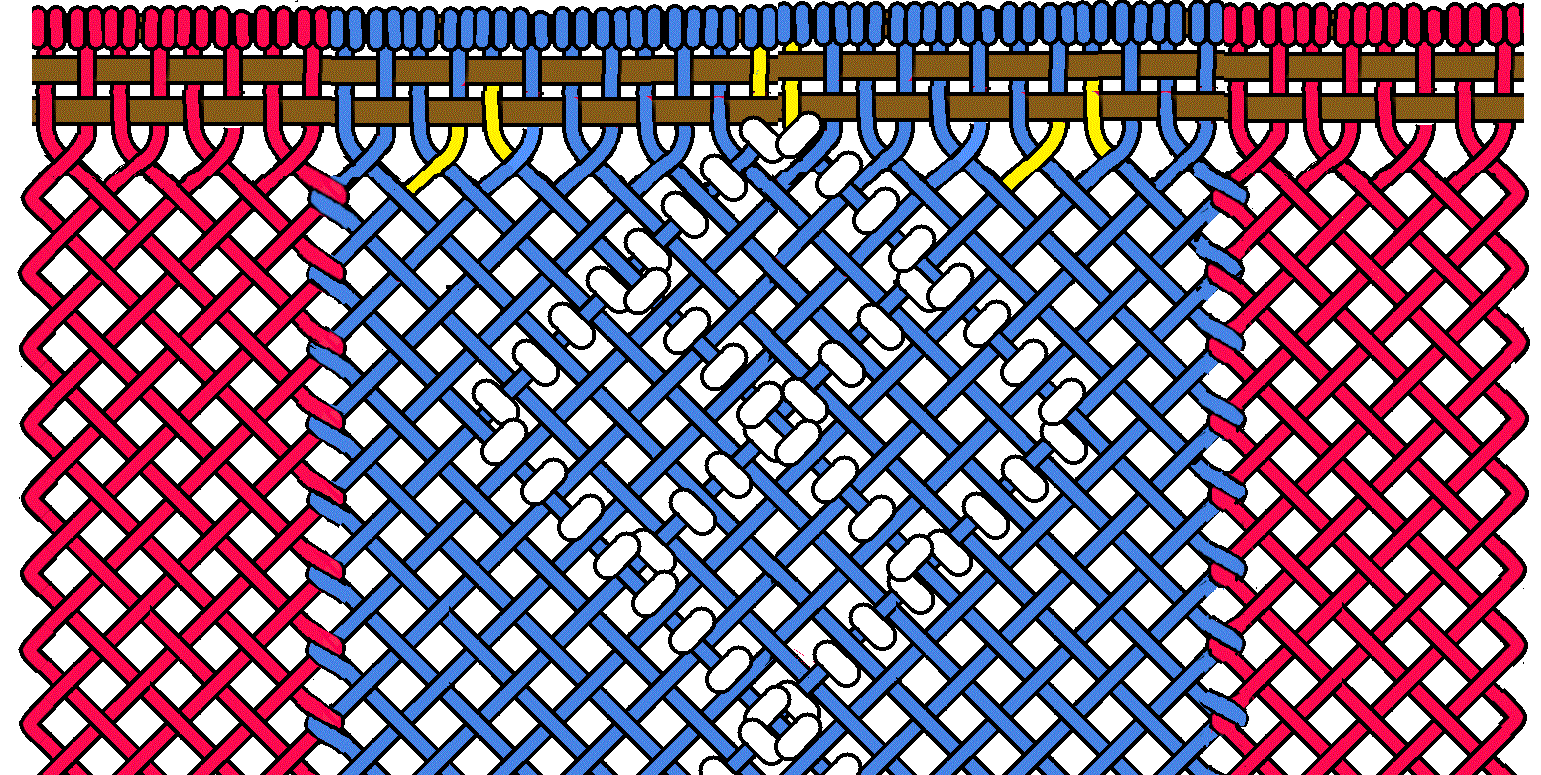``` The table "4 Bead Matrix" shows that the next set of strands that can be used as a 4 bead matrix is a set of 24 strands. 24 is a multiple of 4, the number of beads on each side of the beaded diamond. 24 is also an even number. One half of 24 is 12 which is a multiple of 4, the number of beads on each edge of the beaded diamond. The Middle two strands are identified as carrier strands. ``` ``` The table of values indicates 6 carrier strands, therefore there are 2 additional carrier strands on each side of the middle carrier strands . If you count the number of strands in each layer of the shed on either side of the middle carrier strand, you will find that on one side of each layer there are 6 strands. 6 contains 1 set of 4. Therefore one additional carrier strand can be added to that side of each layer of the shed. The other side of each layer of the shed has 5 strands. 5 contains 1 set of 4. Therefore one additional carrier strand can be added to that side of each layer of the shed. ```
 Planning a Matrix Based Beading Pattern ``` The first thing to do is select a set of strands. Use the rules and the table of values to decide on the width of the matrix. [note] when using worsted weight yarn 17 strands is about 1 inch of the finish width. This value can vary depending on the brand of yarn used. Using the value of 17 strands per inch a 3 inch wide sash would require about 51 strands. If each border is made up of a set of 8 strands, that leave a set of 35 strands for the body of the sash. If a 3 bead matrix is to be used you would refer to the table of values for a 3 bead matrix and choose a set of strands that is larger then 35. The set of 36 strands would the closest set. Next, on a printed copy of the openface grid, outhline a set of 36 strands. Then Identify the carrier strands by tracing over them. This can also be done by downloading a copy of the openface grid to a computer and using a graphics program. When the basic matrix is set up, a beading design can be worked out by marking the location of the beads on the matrix graphic. ``` Basic 3 Bead Matrix Setup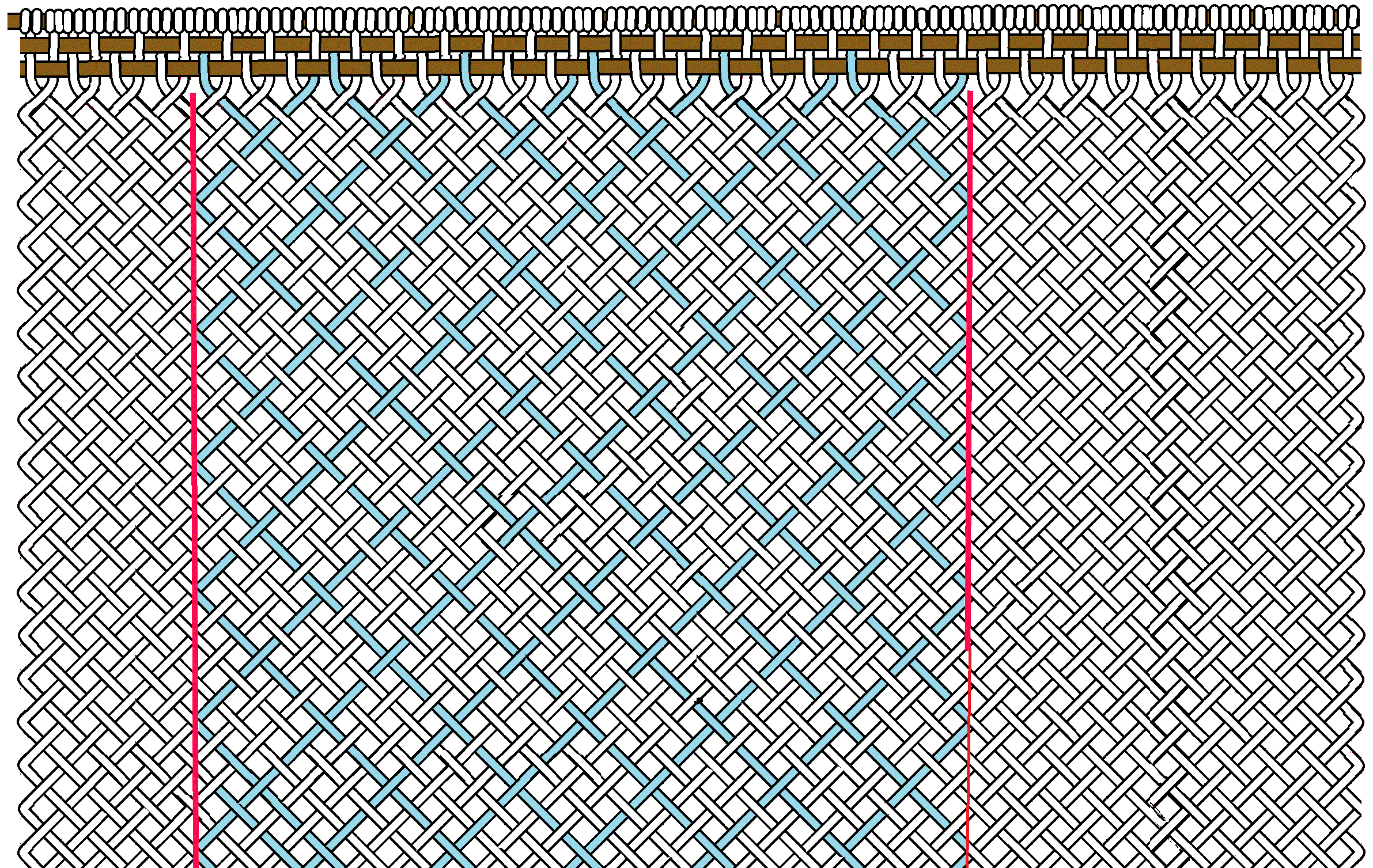Basic 3 Bead Matrix Setup With Bead Designe Example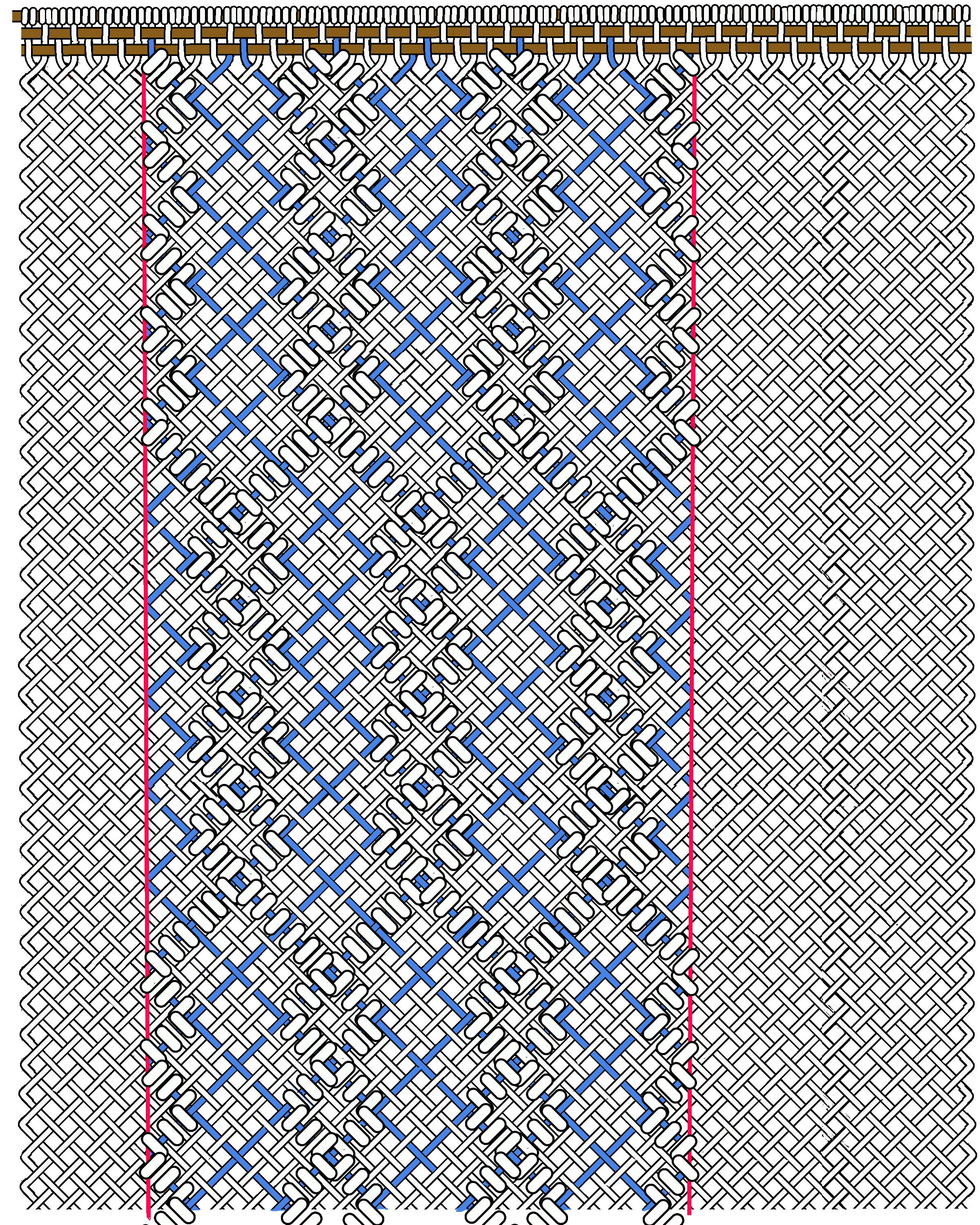Openface Master Grid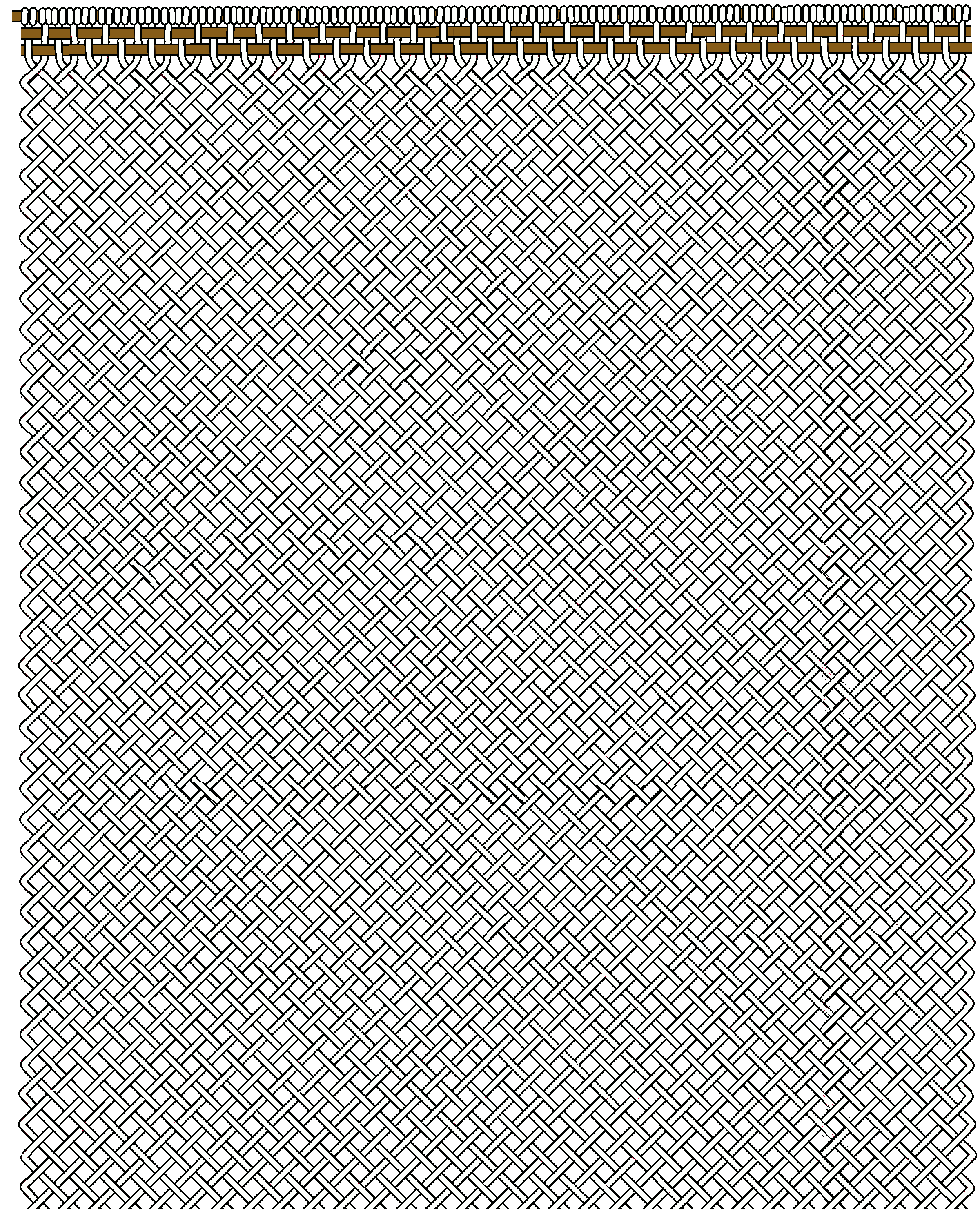"Download Openface Master Grid"